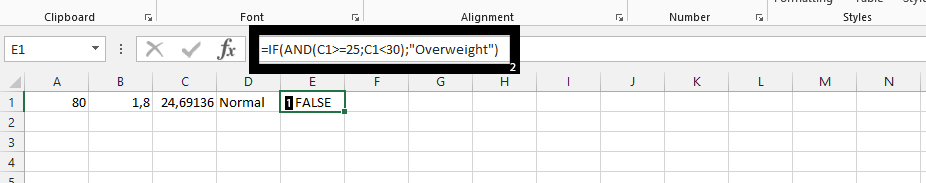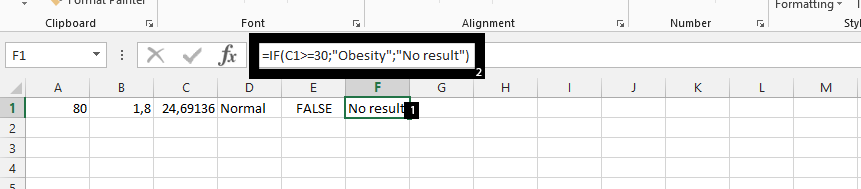## BMI calculator in Excel

The calculation of BMI (Body Mass Index) is purposely meant to know if the person has normal, overweight, or obesity weight. This is possibly done by certain components that make the BMI calculation, a possibility. We will create the calculator together, and even calculate it. Display the weight (1) and height (2) of the person in kilogram and meter.On an empty cell, example C1, click on it (1), divide the weight by height, and add ^2 (2), and press enter.Click on another empty cell (D1) (1), and type =IF(AND(C1>=18.5,C1<=25),”Normal”) (2).Click on another empty cell (E1) (1), and type in what is showing in 2 in the picture below.Click on another empty cell (1), and type in number 2.In conclusion, if it happens that the person is below 18.5, then such person is under weight, and the 80 is in kilogram, while 1.8 was in meter, which means the person is 180cm in height.

## Template

```Further reading: